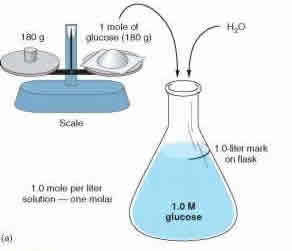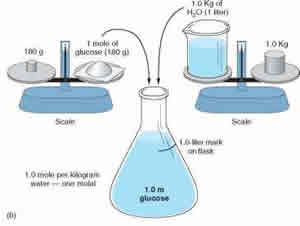# Difference between Molarity and Molality

##### Key Difference: Molarity, also known as molar concentration, measures the number of moles of a substance present in per liter of solution. Molarity is denoted with a capital M. Molality measures the number of moles of a substance present in per kilogram of solvent. Molality is denoted with a lower case m.The term ‘molarity’ and ‘molality’ are two terms that are commonly heard in chemistry. These are two units that are used for measuring the concentration of solutions. The concept of molarity and molality are very confusing for people that are just learning these terms as they seem similar and are both used as measurements. However, these two terms differ in how they measure a particular concentration.

Both of the concepts base their calculations on how many moles are present in the current solution. A solution can be defined as a homogeneous mixture, which means two or more components have been mixed to the point that it cannot be distinguished by the naked eye. These two concepts require understanding moles as it determines the number of moles present in the solution. The theory of moles was discovered by Italian scientist, Amedeo Avogardo.

In 1811, Avogardo proposed that the volume of gas, at a given temperature and pressure, is proportional to the number of atoms or molecules regardless of its nature. This concept is known as the Avogardo constant. It is defined as the number of constituent particles (usually atoms or molecules) in one mole of a given substance. In laymen terms, one mole of a substance would represent the number of atoms and molecules present in the element. For example: Oxygen has an atomic weight of 16, this would represent the mass of per mole of oxygen. So, one mole of oxygen would have a mass of 16 grams. According to Avogardo, one mole of oxygen would have the same number of atoms as one mole of hydrogen. However, they can differ in weight.

Molarity and molality measures the concentration using two different approaches. Molarity, also known as molar concentration, measures the number of moles of a substance present in per liter of solution. Molarity is denoted with a capital M. So a solution with 1.0M would state that it contains 1 mole of solute per liter of solution. For example: A solution of water and sugar. If the solution is measured as 1.0 M, then the solution has 1 mole of sugar per liter of solution present. Molarity is also known as amount-of-substance concentration, amount concentration, substance concentration or simply concentration. Molarity can be calculated using the formula: M = mol (# of moles) / L (volume of solution in liters).Molality measures the number of moles of a substance present in per kilogram of solvent. Molality is denoted with a lower case m. So a solution with 1.0m would state that it contains 1 mole of solute per kilogram of solvent. Let’s differentiate solute from solvent. Solute is the substance that is being dissolved into another substance. A solvent is a substance that dissolves the solute. So, if water and sugar are being mixed together, sugar would be the solute and water the solvent. The mixed sugar water would be the solution. Now, in molality, it is of utmost importance that the moles are divided by the mass of the solvent and not the solution. Molality can be calculated using the formula: m = mol (# of moles) / KG (mass of solvent in kg).

Why the difference? Well, both are used when dealing with temperature. If a solution is going to change in temperature then molality is used. But for solutions that will remain at constant temperature molarity is used. This is because as the temperature rises or falls, the volume of the solution changes and this directly affects the molarity. Hence, molality is used to calculate concentration.

Image Courtesy: genuardis.net